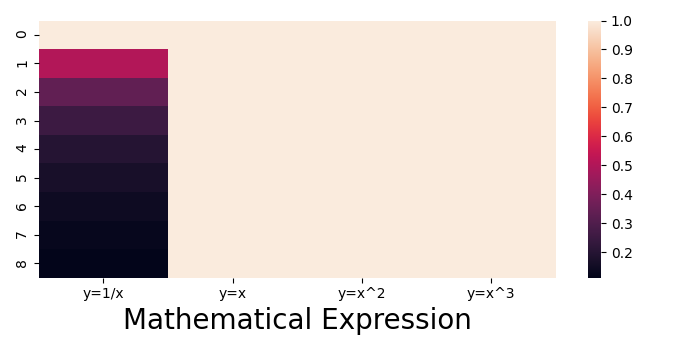# Auto adjust font size in Seaborn heatmap using Matplotlib

To adjust font size in Seaborn, we can take followig steps−

• Create a dictionary with some mathematical expressions
• Create a dataframe using Pandas data frame.
• Create a heatmap using heatmap() method.
• To adjust the font size in Seaborn heatmap, change the fontsize value.
• To display the figure, use show() method.

## Example

import numpy as np
import seaborn as sns
from matplotlib import pyplot as plt
import pandas as pd
plt.rcParams["figure.figsize"] = [7.00, 3.50]
plt.rcParams["figure.autolayout"] = True
d = {
'y=1/x': [1 / i for i in range(1, 10)],
'y=x': [i for i in range(1, 10)],
'y=x^2': [i * i for i in range(1, 10)],
'y=x^3': [i * i * i for i in range(1, 10)]
}
df = pd.DataFrame(d)
ax = sns.heatmap(df, vmax=1)
plt.xlabel('Mathematical Expression', fontsize=16)
plt.show()



## Output